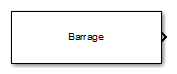# Barrage Jammer

Barrage jammer interference source

## Library

•## Description

The Barrage Jammer block generates a wideband noise-like jamming signal.

## Parameters

Specify the effective radiated power (ERP) in watts of the jamming signal as a positive scalar.

Source of number of samples per frame

Specify the source for number of samples per frame as `Property` or ```Derive from reference input port```. When you choose `Property`, the block obtains the number of samples from the Number of samples per frame parameter. When you choose ```Derive from reference input port``` the block uses the number of samples from a reference signal passed into the `Ref` input port.

Number of samples per frame

Specify the number of samples in the jamming signal output as a positive integer. The number of samples must match the number of samples produced by a signal source. This parameter appears only when Source of number of samples per frame is set to `Property`. As an example, if you use the Rectangular Waveform block as a signal source and set its Output signal format to `Samples`, the value of Number of samples per frame should match the Rectangular Waveform block's Number of samples in output parameter. If you set the Output signal format to `Pulses`, the Number of samples per frame should match the product of Sample rate and Number of pulses in output divided by the Pulse repetition frequency.

Simulate using

Block simulation method, specified as `Interpreted Execution` or ```Code Generation```. If you want your block to use the MATLAB® interpreter, choose `Interpreted Execution`. If you want your block to run as compiled code, choose `Code Generation`. Compiled code requires time to compile but usually runs faster.

Interpreted execution is useful when you are developing and tuning a model. The block runs the underlying System object™ in MATLAB. You can change and execute your model quickly. When you are satisfied with your results, you can then run the block using ```Code Generation```. Long simulations run faster than they would in interpreted execution. You can run repeated executions without recompiling. However, if you change any block parameters, then the block automatically recompiles before execution.

When setting this parameter, you must take into account the overall model simulation mode. The table shows how the Simulate using parameter interacts with the overall simulation mode.

When the Simulink® model is in `Accelerator` mode, the block mode specified using Simulate using overrides the simulation mode.

Acceleration Modes

 Block Simulation Simulation Behavior `Normal` `Accelerator` `Rapid Accelerator` `Interpreted Execution` The block executes using the MATLAB interpreter. The block executes using the MATLAB interpreter. Creates a standalone executable from the model. `Code Generation` The block is compiled. All blocks in the model are compiled.

## Ports

Note

The block input and output ports correspond to the input and output parameters described in the `step` method of the underlying System object. See link at the bottom of this page.

PortDescriptionSupported Data Types
`Ref`Reference signal inputDouble-precision floating point
`Out`Jammer outputDouble-precision floating point

## Version History

Introduced in R2021a